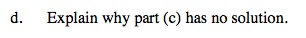Home > A2C > Chapter 8 > Lesson 8.1.4 > Problem8-69

8-69.
1. Solve each equation. Homework Help ✎

1.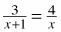2.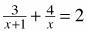3.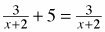4. Explain why part (c) has no solution.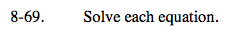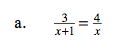Multiply both sides of the equation by x(x + 1).

x = −4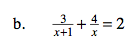See part (a).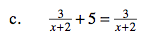Look at the equation carefully before you try to solve it.

No solution

$\text{Substitute }a=\frac{3}{x+2}$